# Review — Deep Captioning with Multimodal Recurrent Neural Networks (m-RNN)

## Using CNN+RNN for Captioning, Generate Sentence from Image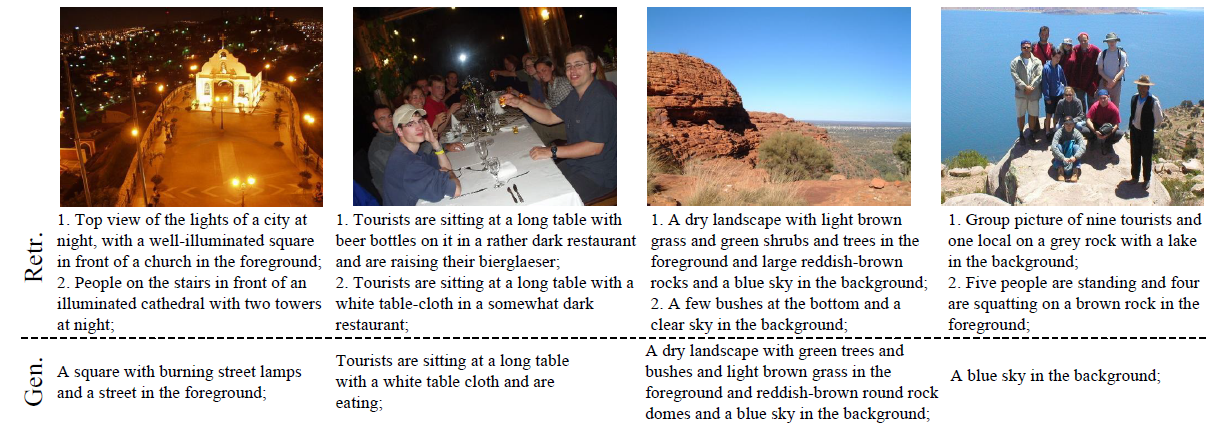Examples of the generated and two top-ranked retrieved sentences given the query image from IAPR TC-12 dataset

In this story, Deep Captioning with Multimodal Recurrent Neural Networks (m-RNN), by University of California, and Baidu Research, is reviewed. In this paper:

• A multimodal Recurrent Neural Network (m-RNN) model is designed for generating novel image captions.
• The model consists of two sub-networks: a deep recurrent neural network for sentences and a deep convolutional network for images, interacts with each other.

This is a paper in 2015 ICLR with over 1100 citations. (Sik-Ho Tsang @ Medium)

# Outline

1. Simple Recurrent Neural Network (RNN)
2. Multimodal Recurrent Neural Network (m-RNN)
3. Training the m-RNN
4. Experimental Results

# 1. Simple Recurrent Neural Network (RNN)

• The simple RNN has three types of layers in each time frame, the input word layer w, the recurrent layer r and the output layer y.
• y(t), the output at time t, can be calculated as follows:
• where x(t) is a vector that concatenates w(t) and r(t-1), f1(:) and g1(:) are element-wise sigmoid and softmax function respectively, and U, V are weights which will be learned.

# 2. Multimodal Recurrent Neural Network (m-RNN)

• m-RNN has five layers in each time frame: two word embedding layers, the recurrent layer, the multimodal layer, and the softmax layer.

## 2.1. Word Embedding

• The two word embedding layers embed the one-hot input into a dense word representation. It encodes both the syntactic and semantic meaning of the words.

## 2.2. Recurrent Layer

• After the two word embedding layers, there is a recurrent layer with 256 dimensions, which first map r(t-1) into the same vector space as w(t) and add them together:
• where “+” represents element-wise addition and f2(:) is ReLU. ReLU is faster, and harder to saturate or overfit the data than Sigmoid.

## 2.3. Multimodal Layer

• After the recurrent layer, there is a 512 dimensional multimodal layer that connects the language model part and the vision part of the m-RNN model.
• This layer has three inputs: the word-embedding layer II, the recurrent layer and the image representation.
• For the image representation, it can be the activation of the 7th layer of AlexNet or 15th layer of VGGNet.
• The activations of the three layers are mapped to the same multimodal feature space and added together to obtain the activation of the multimodal layer:
• where “+” denotes element-wise addition, m denotes the multimodal layer feature vector, I denotes the image feature. g2(:) is the element-wise scaled hyperbolic tangent function.
• where the scaled hyperbolic tangent forces the gradients into the most non-linear value range and leads to a faster training process than the basic hyperbolic tangent function.

## 2.4. Output Layer

• Both the simple RNN and m-RNN models have a softmax layer that generates the probability distribution of the next word.
• The dimension of this layer is the vocabulary size M, which is different for different datasets.

# 3. Training the m-RNN

• A log-likelihood cost function is used to train the m-RNN. It is related to the Perplexity of the sentences in the training set given their corresponding images. Perplexity is a standard measure for evaluating language model. The perplexity for one word sequence (i.e. a sentence) w1:L is calculated as follows:
• where L is the length of the word sequence, PPL(w1:L|I) denotes the perplexity of the sentence w1:L given the image I. P(wn|w1:n-1, I) is the probability of generating the word wn given I and previous words w1:n-1.
• The cost function of our model is the average log-likelihood of the words given their context words and corresponding images in the training sentences plus a regularization term.
• where Ns and N denotes the number of sentences and the number of words in the training set respectively, Li denotes the length of ith sentences, and θ represents the model parameters.
• The training objective is to minimize this cost function, which is equivalent to maximize the probability of generating the sentences.

# 4. Experimental Results

• 3 tasks are evaluated: Sentences generation; Image retrieval (retrieving most relevant images to the given sentence); Sentence retrieval (retrieving most relevant sentences to the given image).
• 4 benchmark datasets with sentence level annotations: IAPR TC-12, Flickr8K, Flickr30K and MS COCO.

## 4.2. IAPR TC-12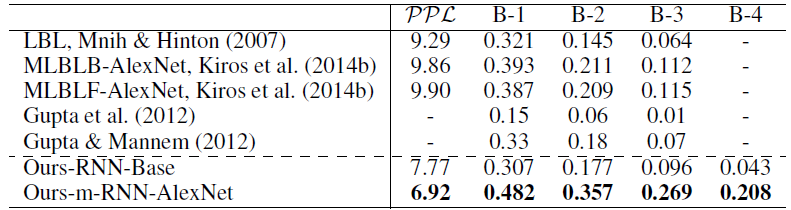Results of the sentence generation task on the IAPR TC-12 dataset. “B” is short for BLEU
• Ours-RNN-Base serves as a baseline method for our m-RNN model. It has the same architecture as m-RNN except that it does not have the image representation input.
• The baseline method of RNN generates a low perplexity, its BLEU score is low, indicating that it fails to generate sentences that are consistent with the content of images.

m-RNN model performs much better than the baseline.

• R@K is the recall rate of a correctly retrieved groundtruth given top K candidates. Higher R@K usually means better. And the R@K scores with smaller K are more important.
• The Med r is another metric, which is the median rank of the first retrieved groundtruth sentence or image. Lower Med r usually means better performance.
• For the retrieval tasks, there are no publicly available results on this dataset.
• The result shows that 20.9% top-ranked retrieved sentences and 13.2% top-ranked retrieved images are groundtruth.

## 4.3. Flickr8K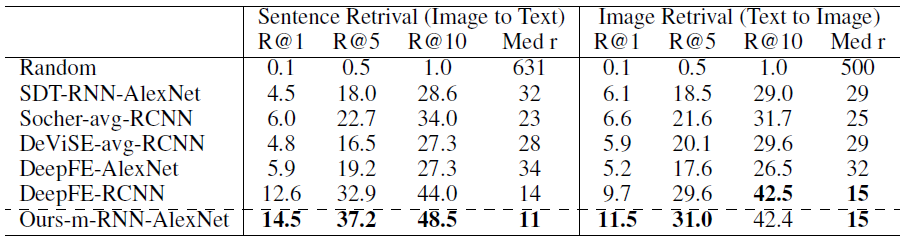Results of R@K and median rank (Med r) for Flickr8K dataset
• “-avg-RCNN” denotes methods with features of the average CNN activation of all objects above a detection confidence threshold.
• Even without the help from the object detection methods, i.e. R-CNN, the proposed method performs better than these methods in almost all the evaluation metrics.
• The PPL, B-1, B-2, B-3 and B-4 of the generated sentences using our m-RNN-AlexNet model in this dataset are 24.39, 0.565, 0.386, 0.256, and 0.170 respectively. (Numbers are not in the above table)

## 4.4. Flickr30K & MS COCO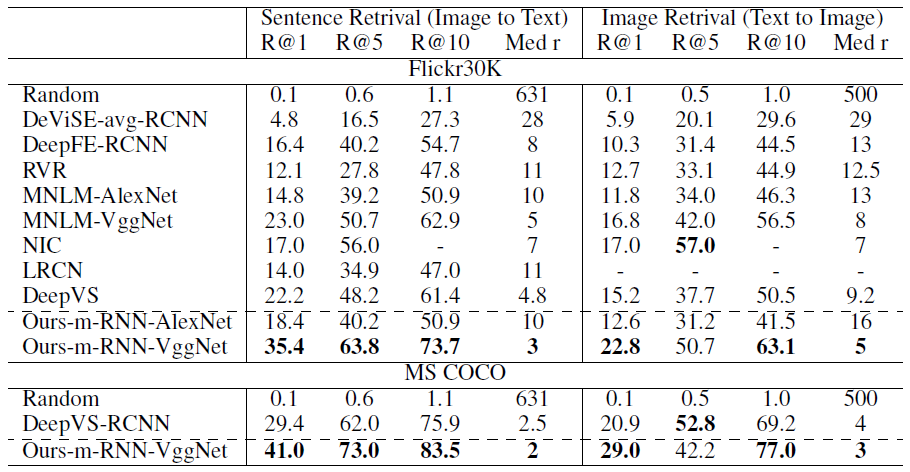Results of R@K and median rank (Med r) for Flickr30K dataset and MS COCO dataset
• For the retrieval tasks, m-RNN-VGGNet performs the best for nearly all evaluations.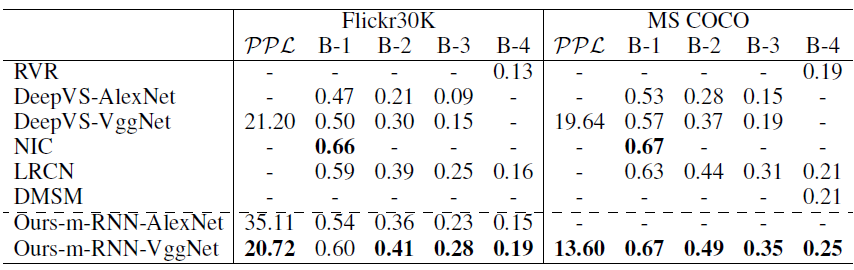Results of generated sentences on the Flickr 30K dataset and MS COCO dataset
• For the sentence generation tasks, m-RNN-VGGNet performs the best for nearly all evaluations.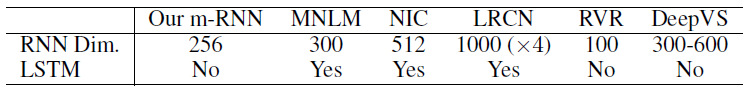Properties of the recurrent layers for the five very recent methods
• Some of the properties of the recurrent layers adopted in the five very recent methods are summarized.
• LRCN has a stack of four 1000 dimensional LSTM layers.

m-RNN achieves state-of-the-art performance using a relatively small dimensional recurrent layer.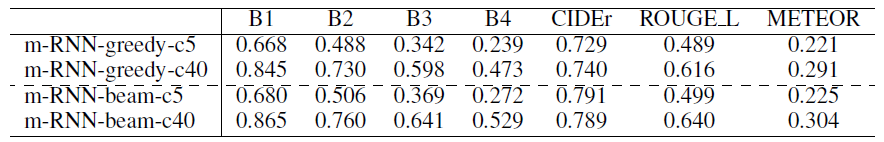Results of the MS COCO test set evaluated by MS COCO evaluation server
• m-RNN model is evaluated with greedy inference (select the word with the maximum probability each time) as well as with the beam search inference.
• “-c5” represents results using 5 reference sentences and “-c40” represents results using 40 reference sentences.

## 4.5. Reranking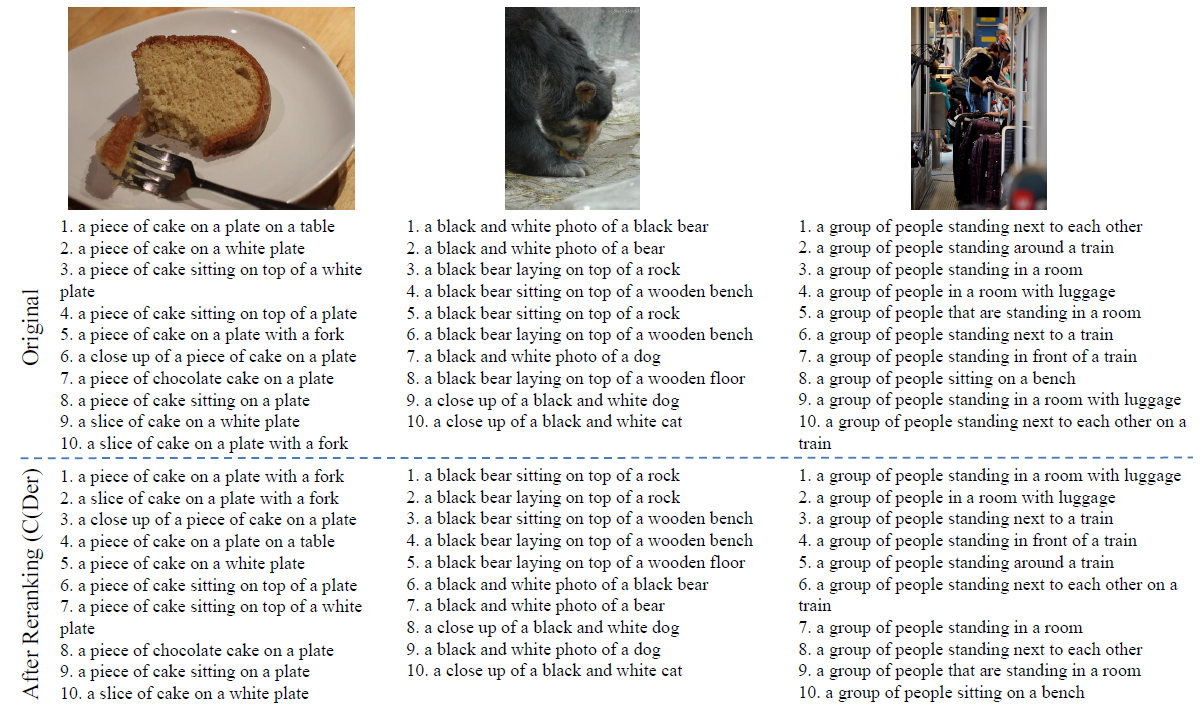The original rank of the hypotheses and the rank after consensus reranking (CIDEr)
• The rank of the ten hypotheses before and after reranking as shown above. Although the hypotheses are similar to each other, there are some variances among them (E.g., some of them capture more details of the images. Some of them might be partially wrong).
• The reranking process is able to improve the rank of good captions.
• (Please feel free to read the paper about the details of reranking. Also, there are still other results in the paper.)

## Reference

[2015 ICLR] [m-RNN]
Deep Captioning with Multimodal Recurrent Neural Networks

## Natural Language Processing (NLP)

2014 [Seq2Seq] [RNN Encoder-Decoder] 2015 [m-RNN]

--

--